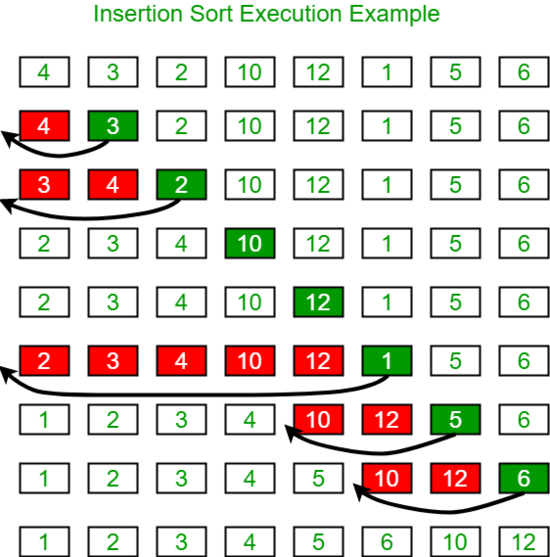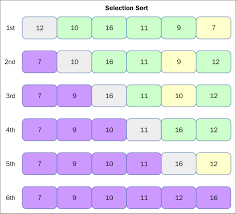# Python实现插入排序和选择排序的方法

更新时间：2019年05月12日 14:54:17   作者：zhuanglog我要评论```def insertion_sort(a):
length = len(a)
if length <=1:
return
for i in range(1,length):
j = i-1
value = a[i]
while j >=0:
if a[j]<value:
a[j+1] = value
break
else:
a[j+1] = a[j]
if j == 0:
a[j] = value
j -=1
print(a)```

return a时间复杂计算为：我们需要将整个数组遍历一遍，所以这一部分为O(n)，在每一次遍历中都要执行一次插入操作，其时间复杂度为O(n)，所以总时间复杂度为O(n2)```def selection_sort(a):
length = len(a)
if length <=1:
return
for i in range(0,length-1):
min_value = a[i]
min_index = i
j = i+1
while j<length:
if a[j] < min_value:
min_value = a[j]
min_index = j
j += 1
a[i],a[min_index] = a[min_index],a[i]```

return a时间复杂度计算：数组长度为n，一共需要寻找n次最小值，每次寻找最小值都要遍历未排序区间一次，其时间复杂度为O(n)，乘以n次为O(n2)